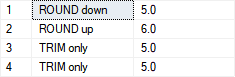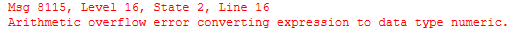T-SQL

# Removing decimal places from a number without rounding

ROUND() is a well-known function in SQL Server. Most of us know only that two parameters can be used like ROUND(99,95, 2). This will do standard mathematical up/down rounding of numbers in the first parameter for the number of decimal places from the second parameter.

But there is also an option to use a third parameter called function specified in BOL as:

• Function is the type of operation to perform.
• Function must be tinyint, smallint, or int.
• When function is omitted or has a value of 0 (default), numeric_expression is rounded.
• When a value other than 0 is specified, numeric_expression is truncated.

Let’s observe this simple example:

```SELECT 'ROUND down', ROUND(5.1, 0) UNION ALL
SELECT 'ROUND up', ROUND(5.9, 0) UNION ALL
SELECT 'TRIM only', ROUND(5.1, 0, 1) UNION ALL
SELECT 'TRIM only', ROUND(5.9, 0, 1)
GO```Using the third parameter with a different value than 0 we have simply trimmed decimal places which can be really handy in case of some specific need for reporting when there is a requirement to report numbers without decimal place but also have a proper value at the total row.

Let me mention one important thing we should remember using the ROUND()  function.

Consider the following example:

```SELECT ROUND(9.9, 0)
GO``````SELECT ROUND(CAST(9.9 AS DECIMAL(3,1)), 0)